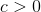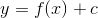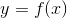## Example Questions

### Example Question #21 : Graphing

SupposeTo obtain the graph of, shift the grapha distance ofunits              .

Up and right

Downwards

Upwards

To the left

To the right

Upwards

Explanation:

There are four shifts of the graph y = f(x):

y = f(x) + c shifts the graph c units upwards.

y = f(x) – c shifts the graph c units downwards.

y = f(x + c) shifts the graph c units to the left.

y = f(x – c) shifts the graph c units to the right.